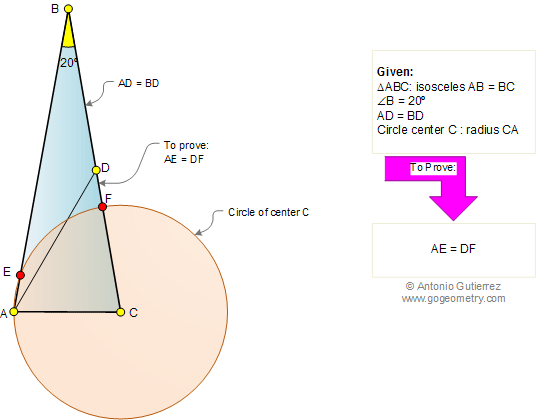Problem 358. Isosceles triangle 80-80-20, Circle, Angles, Congruence. Level: High School, College, SAT Prep. In the figure below, triangle ABC is isosceles with AB = BC and angle B = 20°. The cevian AD is equal to BD and the circle of center C and radius equal to CA cut AB and BC at points E and F, respectively. Prove that AE = DF. Try to use elementary geometry (Euclid's Elements.)Recent Additions